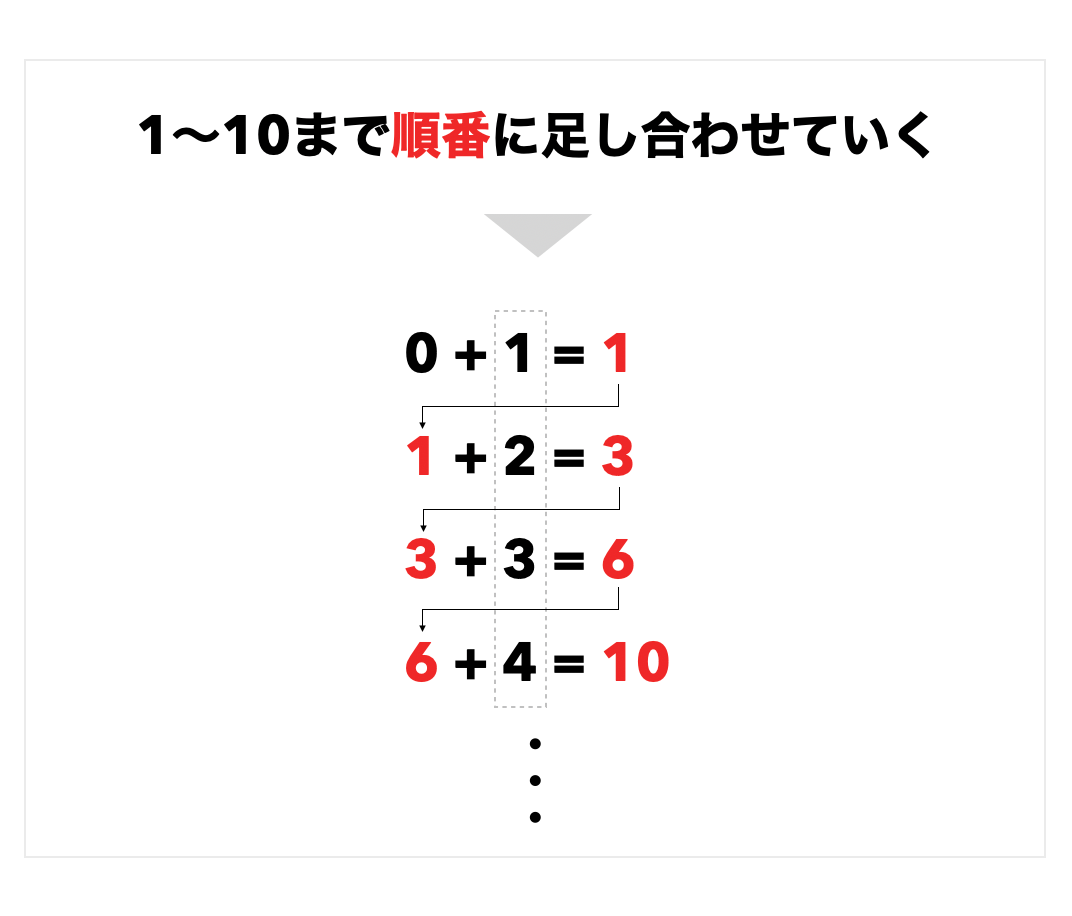# [Ruby] Iterative processing

I am reviewing the drill to strengthen my logical thinking. As a beginner, I would appreciate it if you could let me know if you have any questions. Also this time, I will explain in the order of my thoughts. Please note that this is a way of thinking that novice scholars cannot get a bird's-eye view of, and it is highly possible that it is different from the original way of thinking.

## problem

Write a program that adds the numbers from 1 to 10 in order and outputs the result of adding all at the end to the terminal. Condition: Always use the times statement#### `ruby.rb`

``````
sum = 0      #③

10. times do |i|   #①
sum += i + 1    #②
end

puts sum     #④
``````

## Commentary

This time we will consider in 4 STEP.

(1) First, we want to iterate 10 times, so use times as per the conditions. In the times statement, the number of repetitions is automatically assigned as a numerical value in the variable i, so You can use the variable i to add the number of iterations to the variable. (Any variable in the pipe is OK) However, the value of i for the first time is 0.

#### `ruby.rb`

``````
10. times do |i|

end
``````

(2) Write a program that adds the numbers from 1 to 10 in order. Add 2 to 1 Add 3 to the result Add 4 to the result Adding 5 to the result and repeating up to 10 ... Because it is a form of repeatedly adding 1 to 10 to the result part

Result = (previous) result + numerical value

Can be thought of as. Let's give the result a variable called sum. It doesn't have to be sum. I feel like this.

#### `ruby.rb`

``````
sum  = sum + 1
sum  = sum + 2
sum  = sum + 3   #Lasts up to 10
``````

The numeric part defined i in the times statement. Furthermore, using the self-assignment operator, it can be expressed as follows.

#### `ruby.rb`

``````
sum += i
``````

And note here that the first number of ** i is 0 as I wrote in ①. ** ** If this is left as it is, the first calculation result will be 0, so add +1.

#### `ruby.rb`

``````
10. times do |i|
sum += i + 1
end
``````

③ Define sum. At this rate, I get angry with "What is sum!"

#### `ruby.rb`

``````
sum = 0
``````

Let's define it outside the times statement. If you write it inside, it will be added to 0 every time. (The final result will be 10)

④ Finally, it is necessary to output to the terminal.

#### `ruby.rb`

``````
puts sum
``````

is not it. What happens in this case with irb? I think it is very easy to understand if you experiment with.